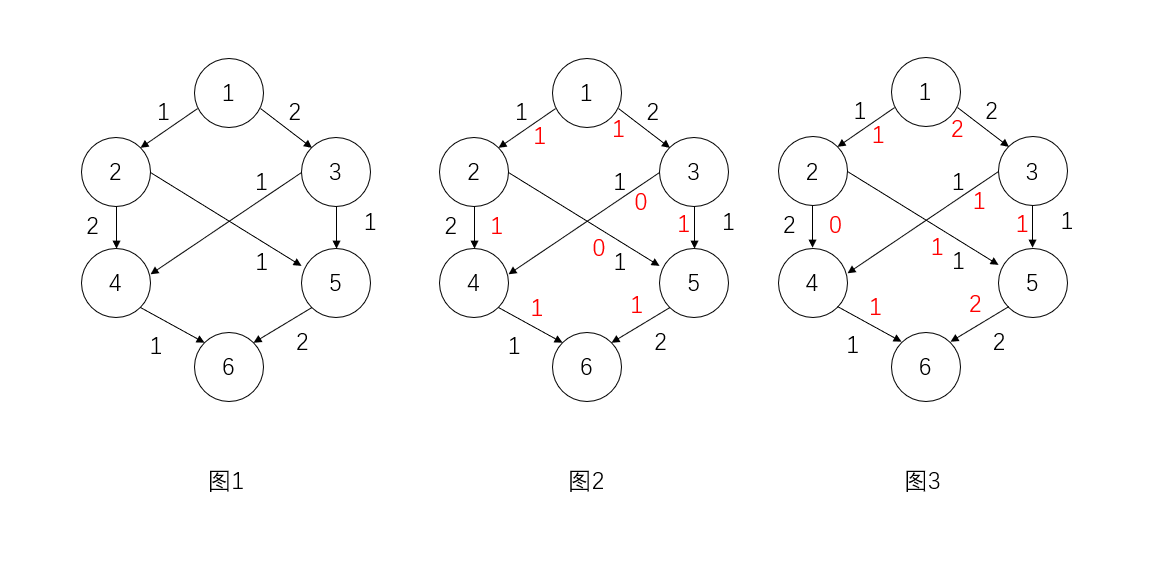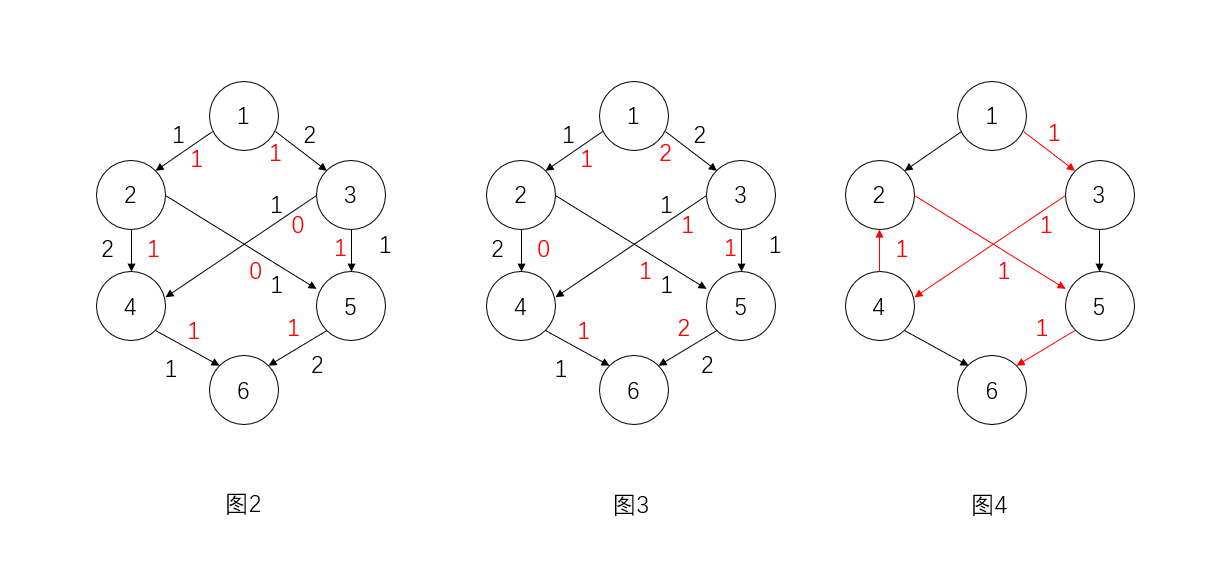# CF630D2F

1. 一条边的两端不同时被染色$V$中任意两点间没有边
2. 没有孤立顶点被染色$V$中的每个点都应当与$E'$中的一条边相连

# Dinic算法

## 问题

$F$较大时影响Ford-Folkerson算法效率的主要因素在于每次dfs可能可以找到许多条路径但是该算法中每次dfs只进行一次增广这就导致增广次数与$F$可能相关一种想法是一次dfs多次增广但这样做能否成功是取决于dfs顺序的于是考虑dfs顺序如何时效率较高呢

## 思路

Dinic算法的核心是每次寻找最短的增广路并进行增广这样做的理由既是依据也是好处每次沿最短增广路增广后最短增广路的长度一定不会减少稍后会给出证明假如有了这一结论每次找到最短增广路的长度时将该长度的增广路[全部增广]然后重新寻找最短增广路其长度至少增1而增广路长度不会超过$|V|$因为增广路是简单路径从而增广次数上界$O(|V|)$

## 证明

1分层图上沿最短增广路增广1次后新图中可能出现一些新的反向边若新图中有增广路$l'$其中的边的来源有两种可能

1边原分层图中的边bfs时经历过的边$\langle u,v\rangle$满足$n_v\leq n_u+1$

2边增广时出现的反向边$\langle v,u\rangle$记原边为$\langle u,v\rangle$那么由于原边在最短增广路中一定满足$n_v=n_u+1$

2假设沿最短增广路增广n次后最短增广路长度不减少当增广$(n+1)$次后若图中无增广路结论成立若图中仍有增广路其中的边可能是

1边增广n次后的图中的边$\langle u,v\rangle$满足$n_v\leq n_u+1$

2边$(n+1)$次增广时出现的反向边$\langle v,u\rangle$记原边为$\langle u,v\rangle$那么由于原边在最短增广路中一定满足$n_v=n_u+1$

## 模板

#include <iostream>
#include <cstdio>
#include <vector>
#include <queue>
#include <cstring>

#define V 5005 //V为顶点最大个数<span class="bd-box"><h-char class="bd bd-beg"><h-inner>，</h-inner></h-char></span>视数据范围而定
#define INF 0x7fffffffffffffff

using namespace std;
typedef long long ll;
struct edge{
ll to, w, rev;
};
vector<edge> G[V];
int num[V], iter[V];
int s, t;//源点和汇点<span class="bd-box"><h-char class="bd bd-beg"><h-inner>，</h-inner></h-char></span>是全局变量

ll max_flow();
ll dfs(ll u, ll f);
void bfs();
void add_edge(ll from, ll to, ll w);

ll max_flow() {
ll f = 0, flow = 0;
while(true) {
memset(iter, 0, sizeof(iter));
bfs();
if(num[t] == -1) return flow;
while(f = dfs(s, INF)) {
flow += f;
}
}
}
ll dfs(ll u, ll f) {
if(u == t) return f;
ll flow;
for(int &i = iter[u]; i < G[u].size(); i++) {
edge &e = G[u][i];//注意是引用<span class="bd-box"><h-char class="bd bd-beg"><h-inner>，</h-inner></h-char></span>需要直接修改原图
if(e.w > 0 && num[u] < num[e.to]) {
if(flow = dfs(e.to, (f > e.w ? e.w : f))) {
//min函数有些编译器不给过<span class="bd-box"><h-char class="bd bd-beg"><h-inner>，</h-inner></h-char></span>所以用三目
e.w -= flow;
//直接修改容量而非同时记录f和w<span class="bd-box"><h-char class="bd bd-beg"><h-inner>，</h-inner></h-char></span>只是为了方便
G[e.to][e.rev].w += flow;
return flow;
}
}
}
return 0;
}
void bfs() {
memset(num, -1, sizeof(num));
queue<int> que;
que.push(s);
num[s] = 0;
while(!que.empty()) {
int u = que.front(); que.pop();
for(int i = 0; i < G[u].size(); i++){
edge &e = G[u][i];
if(e.w > 0 && num[e.to] < 0) {
num[e.to] = num[u] + 1;
que.push(e.to);
}
}
}
}
void add_edge(ll from, ll to, ll w) {
G[from].push_back(edge{to, w, G[to].size()});
G[to].push_back(edge{from, 0, G[from].size() - 1});
}


# 网络流

## 最大流问题### 改进Ford-Fulkerson算法

## 模板

#include <iostream>
#include <cstdio>
#include <vector>
#include <queue>
#include <cstring>

#define V 5005 //V为顶点最大个数<span class="bd-box"><h-char class="bd bd-beg"><h-inner>，</h-inner></h-char></span>视数据范围而定
#define INF 0x7fffffffffffffff

using namespace std;
typedef long long ll;
struct edge {
ll to, w, rev;
};
vector<edge> G[V];
int s, t;//源点和汇点<span class="bd-box"><h-char class="bd bd-beg"><h-inner>，</h-inner></h-char></span>是全局变量
bool vis[V];

ll max_flow();
ll dfs(ll u, ll f);
void add_edge(ll from, ll to, ll w);

ll dfs(ll u, ll f) {
if(vis[u]) return 0;
vis[u] = true;
if(u == t) return f;
for(int i = 0; i < G[u].size(); i++) {
edge &e = G[u][i];//注意是引用<span class="bd-box"><h-char class="bd bd-beg"><h-inner>，</h-inner></h-char></span>需要直接修改原图
if(!vis[e.to] && e.w > 0) {
ll curf = dfs(e.to, (f > e.w) ? e.w : f);
//min函数有些编译器不给过
if(curf > 0) {
e.w -= curf;
//直接修改容量而非同时记录f和w<span class="bd-box"><h-char class="bd bd-beg"><h-inner>，</h-inner></h-char></span>只是为了方便
G[e.to][e.rev].w += curf;
return curf;
}
}
}
return 0;
}
ll max_flow() {
ll maxf = 0;
while(true) {
memset(vis, 0, sizeof(vis));
ll f = dfs(s, INF);
if(f == 0) break;
maxf += f;
}
return maxf;
}
void add_edge(ll from, ll to, ll w) {
G[from].push_back(edge(to, G[to].size(), w));
G[to].push_back(edge(from, G[from].size() - 1, 0));
}